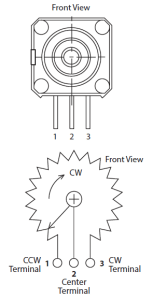# Sigmoid Potentiometer Taper

By paying attention to mistakes, we invest more time and effort to correct them. The result is that you make the mistake work for you.

— Jason Moser

## Introduction

Yesterday, I had a question from a reader on how to develop mathematical formulas for different potentiometer tapers. Normally, I would simply answer the questioner without a separate post, but my solution for this particular question provided a nice illustration of basic coordinate transformations. Since I have not shown any coordinate transformation applications in this blog before, I thought it would be worthwhile to make a post of my response.

There many different names (e.g. "M", "W", "S") assigned to the common potentiometer tapers.  To my knowledge, the taper names vary by vendor. For this post, I will use the taper names as stated in Figure 1 by State Electronics, which the questioner referred to. My work here will focus on the M and W tapers, which are closely related.

My analysis assumes that the potentiometer taper is an actual exponential curve. For ease of manufacture, many potentiometer suppliers approximate the exponential curve using a piecewise linear approximation. For example, Figure 1 shows a W taper that appears to be composed of three linear segments.

I should also mention that I add a constant term to my exponential function to allow my curve fit to pass through zero, which is what happens with real potentiometers –  a true exponential curve, i.e.$\displaystyle y={{e}^{x}}$, would not pass through zero.

## Background

### Potentiometer Construction

This discussion will focus on the common, three-terminal potentiometer. Figure 2(a) shows how State Electronics defines the terminals and Figure 2(b) shows what a potentiometer looks like inside.Figure 2(a): Terminal Definitions. Figure 2(b): Physical Construction of a Three-Terminal Potentiometer (source).

### W Taper

The W taper is sometimes referred to as the antilog taper because it related to the exponential function. Its specific functional form of the potentiometer resistance between terminals 1 and 2 is dictated by the following definition.

The “W” taper attains 20% resistance value at 50% of
clockwise rotation (left-hand).

I should mention that you rarely see the W taper described in terms of an actual function – it resistance versus wiper position is almost always shown as a graph. Remember that these are physical parts and they vary quite a bit from their nominal specifications.

### M Taper

The M taper has a sigmoid shape and its resistance between terminals 1 and 2 is defined in terms of the W taper as follow.

The “M” taper is such that a “W” taper is attained from
either the 1 or 3 terminal to the center of the element.

## Analysis

### W-Taper Characteristic

Figure 3 shows my approach to developing a W taper functional relationship.

### M-Taper Characteristic

Figure 4 shows my approach to developing an M taper functional relationship.

Figure 5 shows my combined plot of the M and W tapers. They are very similar to that shown in Figure 1.

## Conclusion

This post demonstrated how to develop functional relationships for the resistance of two common types of potentiometer tapers. It does seem odd that these functions are never actually stated in the vendor documentation, but hopefully I have alleviated that shortcoming here.

This entry was posted in Electronics. Bookmark the permalink.

### 4 Responses to Sigmoid Potentiometer Taper

1.Gene Mirro says:

For the W taper, what is F1(x,k) ? What is del sub K ? Can you explain how you knew that you needed the F1(x,k) expression? Thanks.

•mathscinotes says:

F1 and del sub K are just part of the setup that Mathcad requires to use its nonlinear curve fit routine. You can do the same calculation in Excel using Solver and minimizing the mean square error between the data and the curve fit.

mathscinotes

2.Boyan Petrov says:

I had 2 free days and nothing else to do.
Inspired by this blog, I think I did it in more 'school view'.
https://www.filedropper.com/audiopots
http://www.filedropper.com/ratpot1
http://www.filedropper.com/potexpm20